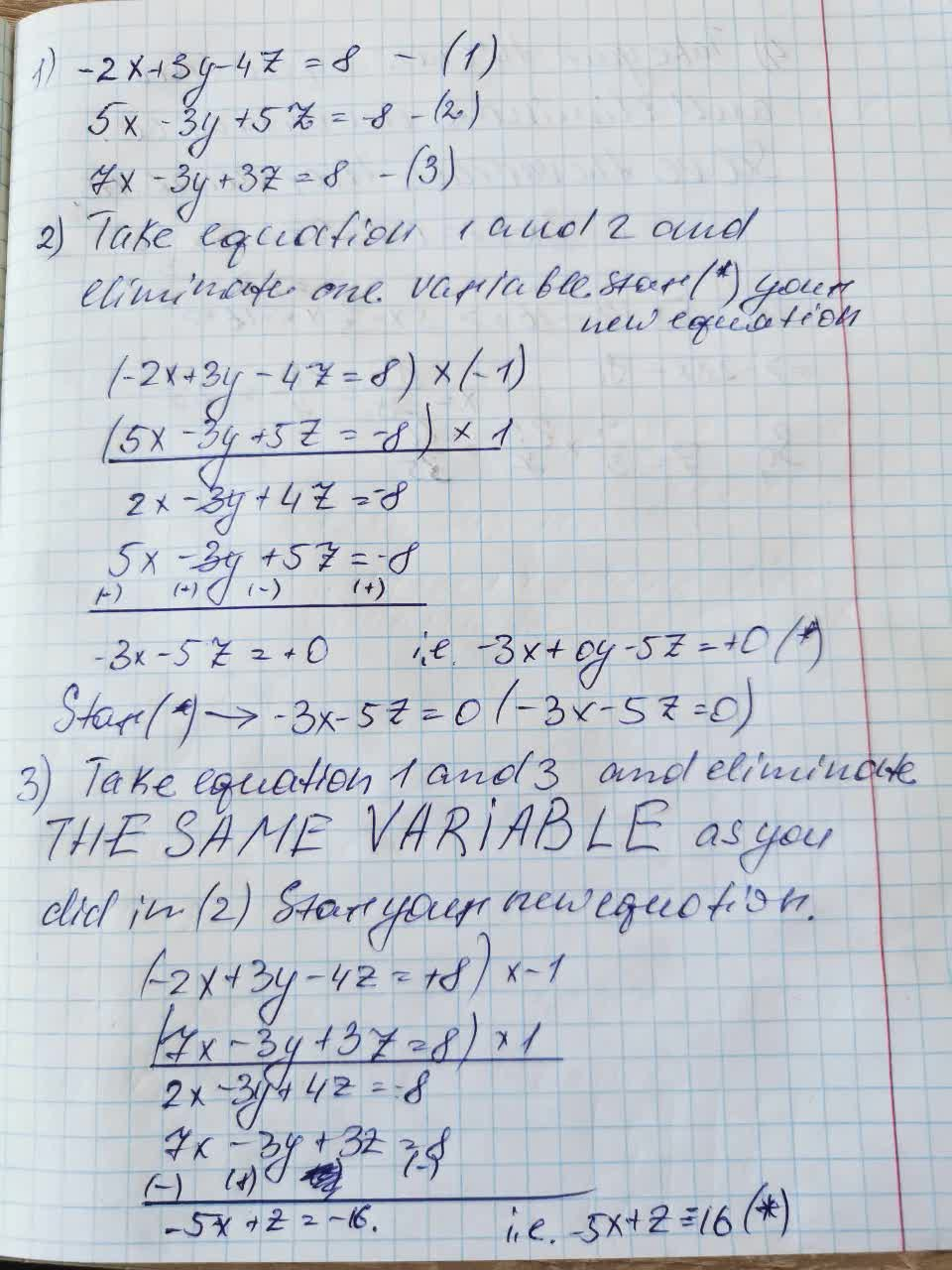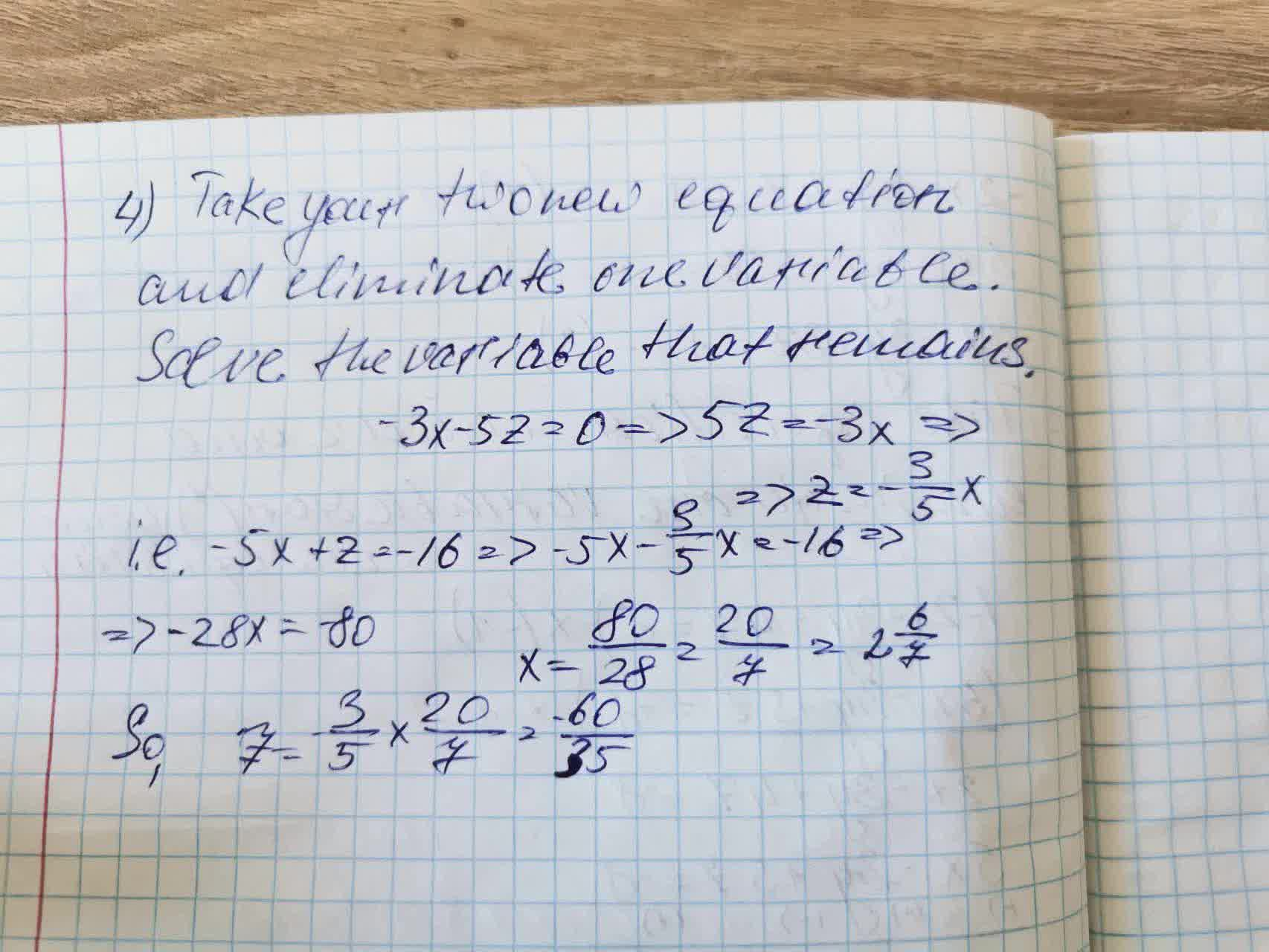Question# Solve the following system of linear equations.-2x+3y-4z=8,5x-3y+5z=-8,7x-3y+3z=8

Equations
ANSWEREDSolve the following system of linear equations
$$\begin{cases}-2x+3y-4z=8\\5x-3y+5z=-8\\7x-3y+3z=8\end{cases}$$